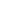# If 10% discount and then 40% discount in succession is equivalent to total of:

a) 40%
b) 30 %
c) 46%
d) 50%
SSC CHSL-2015

 46%. Solution If the first discount is x% and 2nd discount is y% then , Total discount = ( x + y - xy / 100 ) % x = 10% and y = 40% Total discount = [ 10 + 40 – ( 10 x 40) / 100] % = ( 50 – 400 /100 ) % Total discount = 46%
Share :
+
Next
« Prev
Prev
Next »